# 11+ Metric System Conversion Chart Templates

Metric System was first discovered more than two hundred years ago during the French Revolution and even to this day, the Metric system conversion chart is used by many individuals to convert units of measurement without going through the hassles of calculating mathematically. These Metric Conversion Chart Templates especially comes to use because different countries have their own units of measurement. Some of the popular units of the metric system are temperature, pressure, area, volume, height, currency speed and time.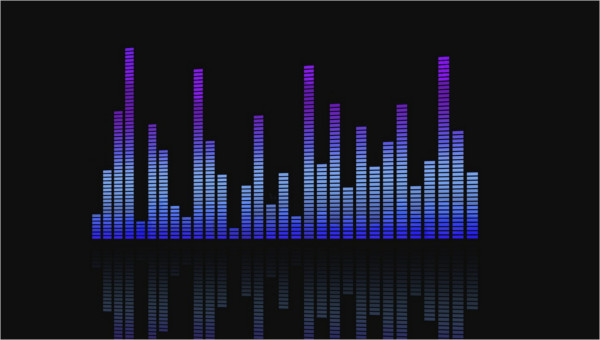## Metric System Conversion Chart For Nurse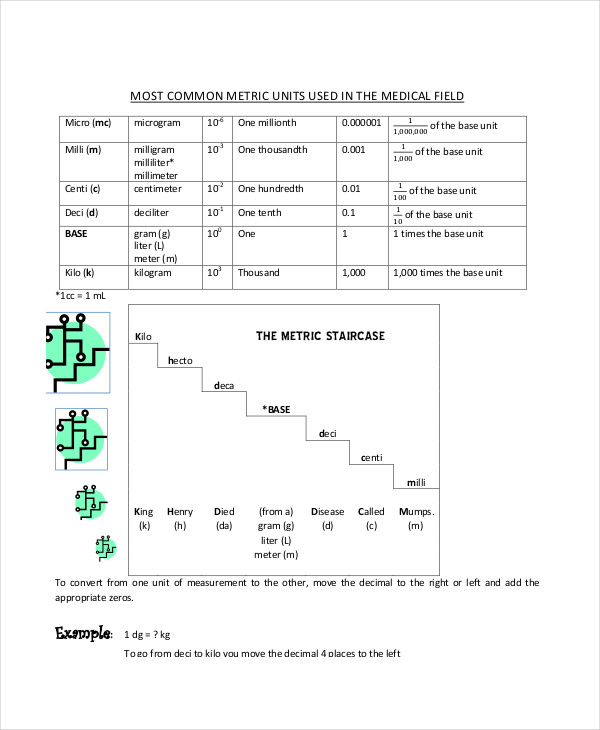laniertech.edu

This conversion Chart Templates can be used by Nurses to measure the height and weight of the patients and for calculation of drug dosage which might vary if they are dealing in solid and liquid medications and as per the nature of the illness of the patient.

## Metric System Conversion Chart For 5Th Grade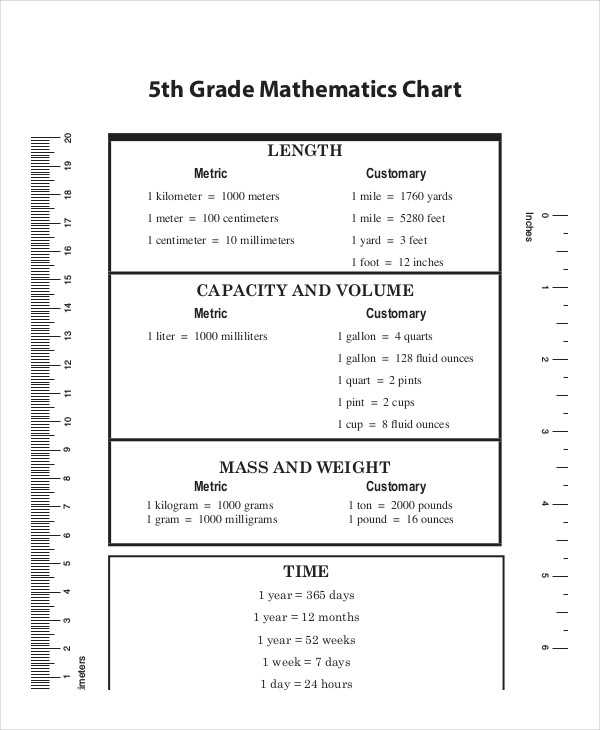store.lonestarlearning.com

For primary school students, this simple metric system might comprise only of length, breath and volume or of speed and time so that they are able to learn the conversion math step by step.

## Metric System Conversion Chart 4Th Grade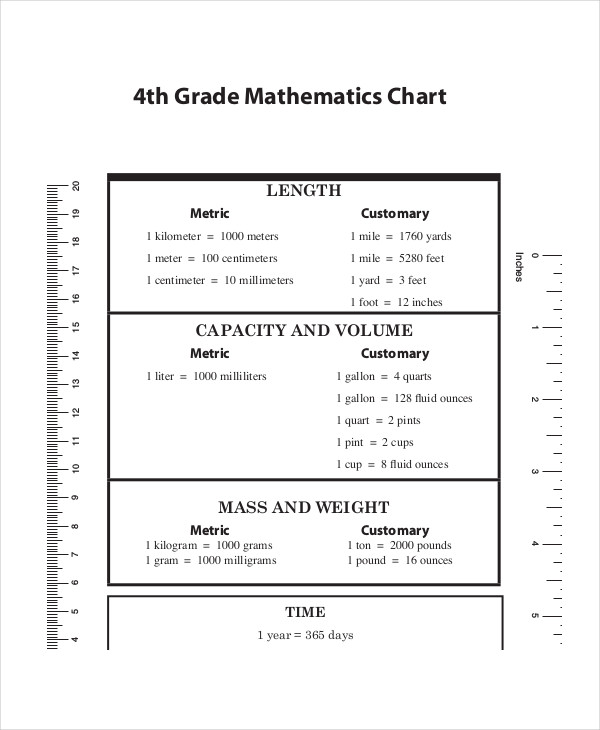store.lonestarlearning.com

Apart from learning basic mathematics, the 4th graders of primary school can be acquainted with this chart so that they understand the mechanism of unit conversion and are able to solve simple conversion sums.

## Metric System Conversion Chart Worksheet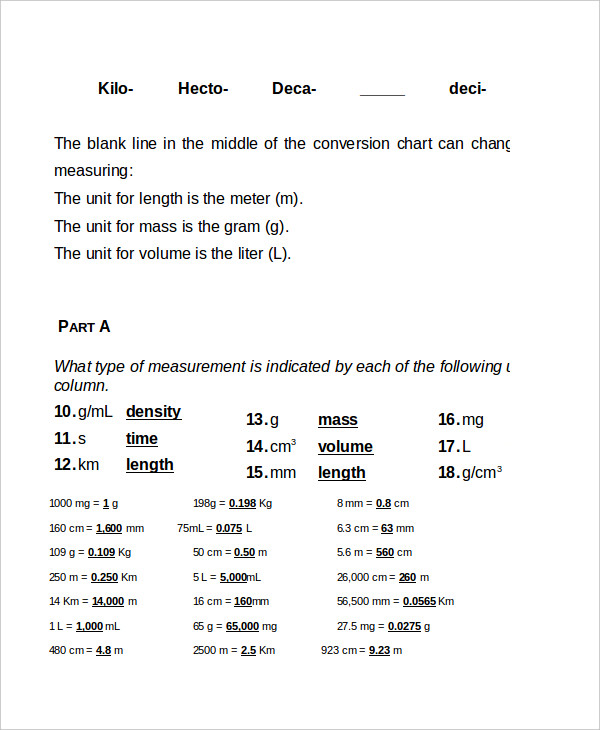trinitypride.org

You can furnish this metric conversion chart worksheet to students who have just learned the Metric System conversation process and require their knowledge and understanding to be put to test.

## Volume Conversion Chart For Metric System

The volume conversion chart comprises of units such as ounce, pint, quart and gallon and makes calculating much simpler and easier. This chart can be specifically used to calculate liquid matter.

## Sample Metric Conversion Chart Template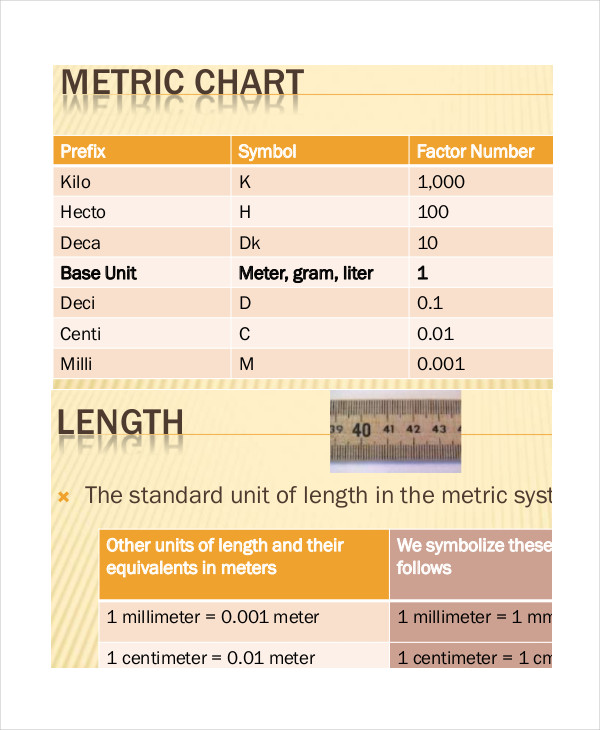sradai.tripod.com

## Metric Unit Conversion Chart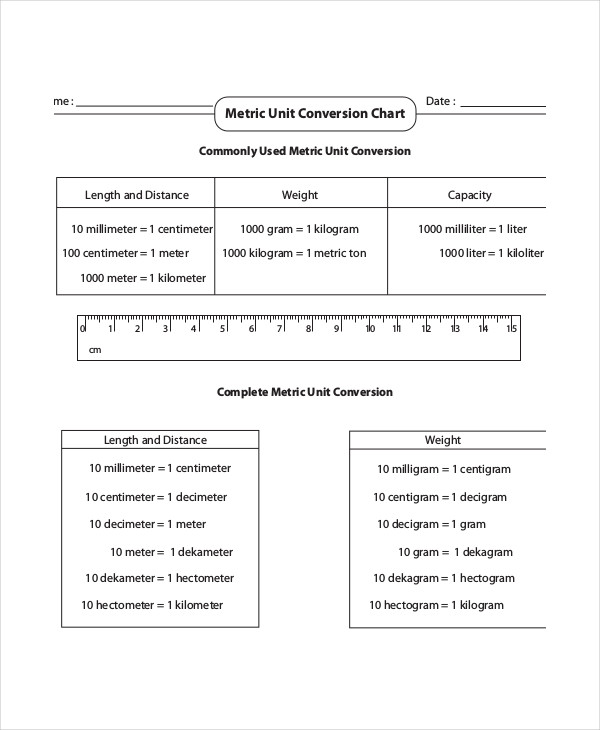mathworksheets4kids.com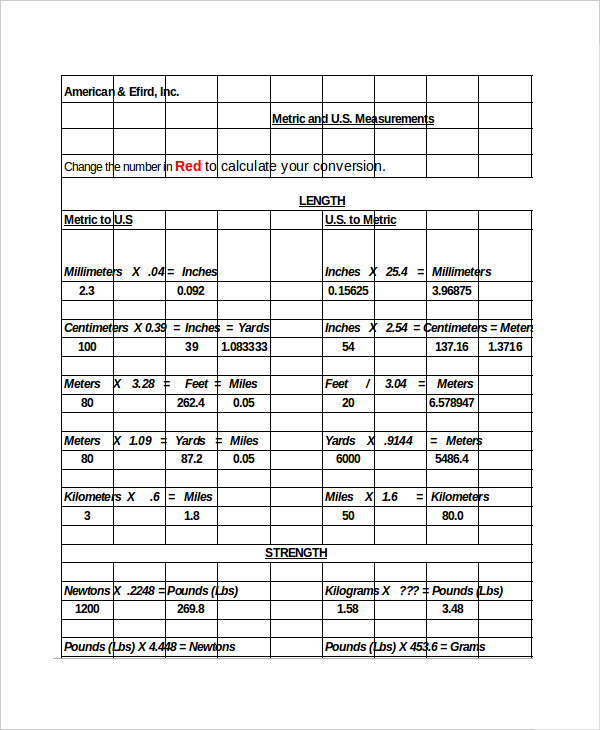amefird.com

## Metric Conversion Chart for Kids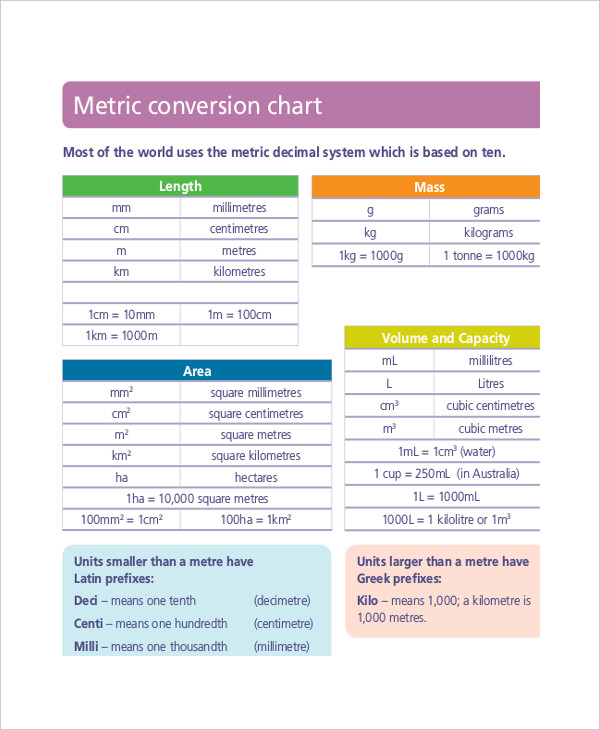det.nsw.edu.au

## Height & Weight Metric Conversion Chart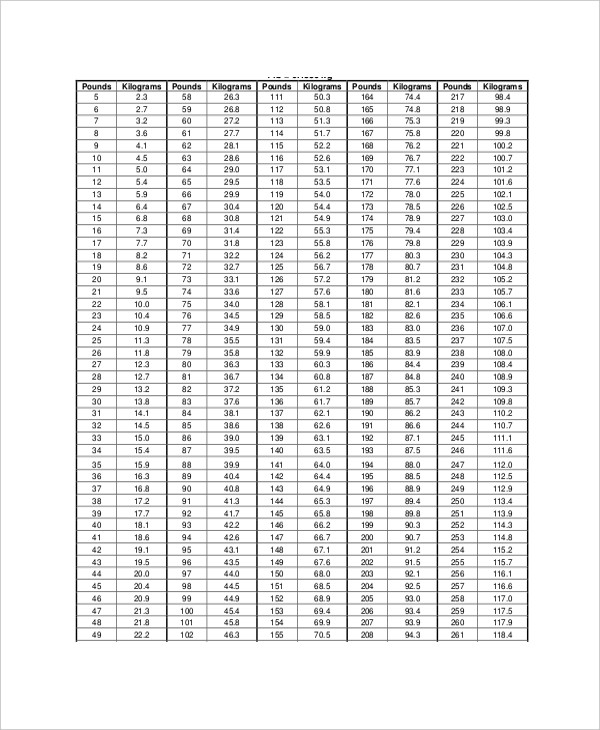calgarylabservices.com

## Simple Metric Conversion Chart Template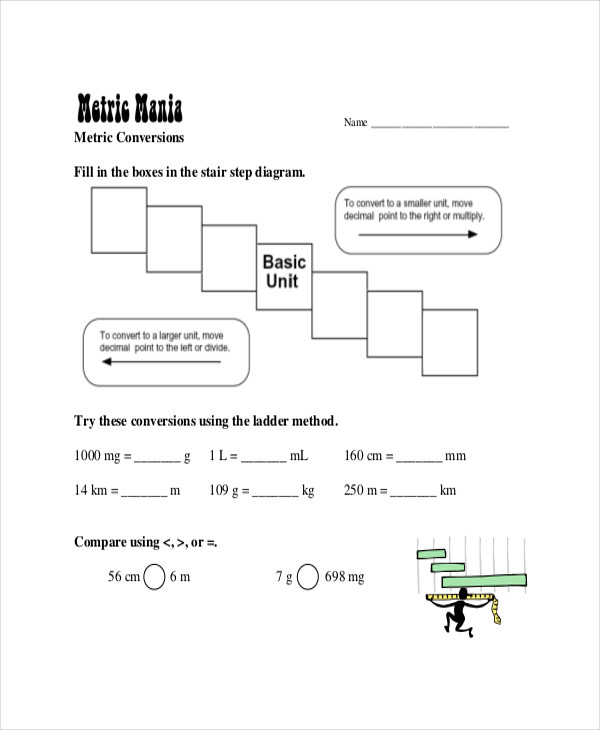sciencespot.net

## Weight Metric Conversion Chart Template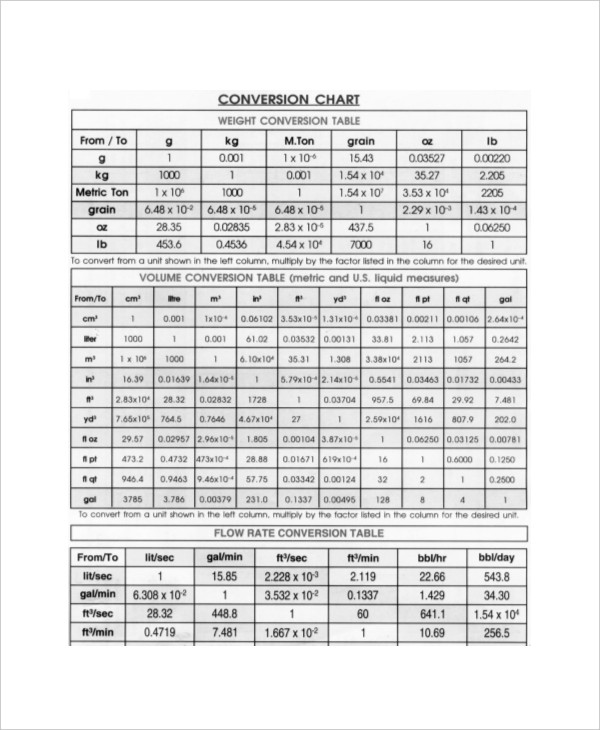blulux.com

### > How to use the Metric System Conversion Chart?

Metric System conversion calculator makes complex mathematical calculations easy. It saves time and assists the users to make conversions comfortably. This chart finds usage in myriad professions for converting units such as length, breadth, area, volume, temperature and light. Besides, the specialized charts which concentrate on only specific units are the ultimate solutions for numerous professionals. The best part about using the Metric system conversion chart is that you do not have to understand the complex math to make a few conversions yourself – simply glance at this chart to pick the unit and the corresponding value can help you make a couple calculations easily. You can also see Word Metric Conversion Chart Templates.

### > What are the Benefits of the Metric System Conversion Chart?

The metric system or International System of Units (SI) is based on seven primary units for quantities that are mutually independent. The quantities are mass (kilogram), temperature (kelvin), electric current (ampere), length (meter), time (second), substance (mole) and light(candela).

Using a conversion chart ensures that you do not have to spend a lot of time in doing prolonged calculations in deriving the value of a conversion unit which you can otherwise obtain by simply referring to the chart. Irrespective of whether you are a mathematician, this handy chart can be helpful to everyone, even in your kitchen to measure ingredients for a new recipe. You can also see Height Weight Chart Templates.

Kilo and Mili are the most prevalent units of the Metric Conversion chart used by people across the world. However, the metric conversion chart finds a place in the school curriculum of the primary school students for them to understand and get used to calculating unit conversions from an early age. This chart is user-friendly and even if you are not competent in Mathematics, you can still use it at ease.